# 64 in Binary

In this mini-lesson, we will learn how to convert 64 to binary by understanding the rules for converting numbers from decimals to binary and how to apply them while solving problems. We will also discover interesting facts around them.

Humans can communicate with each other by means of languages.

Americans communicate with each other in English, French people talk in French, while Indians have their conversations in Hindi or English.

But have you ever thought of how two machines talk to each other?

Or how humans communicate with machines?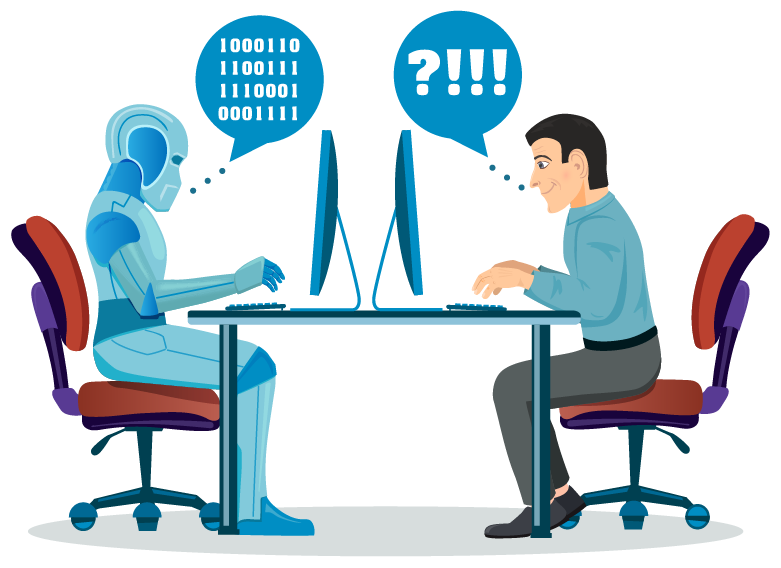Machines can only understand one language, i.e., binary language.

Machines convert every form of inputs from the user to binary language and then perform the required task.

For example, if you write "Hello World" on your keyboard, your computer first converts this into a binary language and then displays your text on the screen.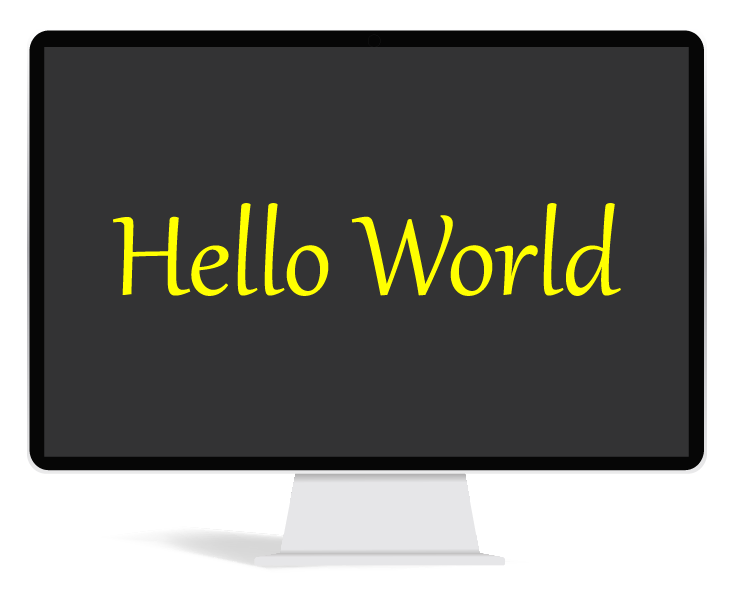The binary language contains only two numbers, 0 and 1

These numbers 0 and 1 are called bits.

## Lesson Plan

 1 What Is Meant by 64 In Binary? 2 Important Notes 3 Solved Examples 4 Challenging Questions 5 Interactive Questions

## What Is Meant by 64 In Binary?

If someone asks you to write the number 64, you simply write it like this – 64

But what if you are asked to write 64 in decimal form?

$$64_{10}$$ is the decimal representation of 64, where 10 is referred to as the base of the number.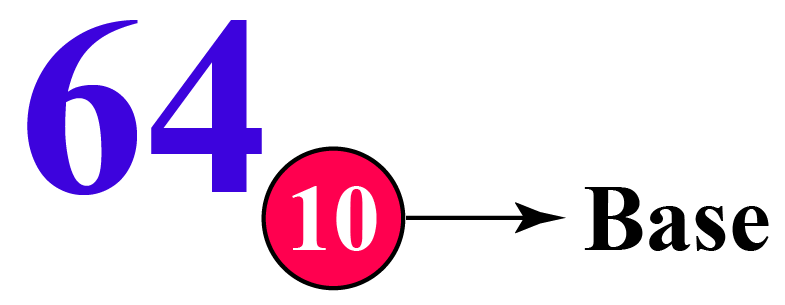Converting 64 from decimal to binary means to write or represent $$64$$ using $$2$$ bits only, i.e., $$0$$ and $$1$$

For converting 64 from decimal to binary, we need to change the base from $$10$$ to $$2$$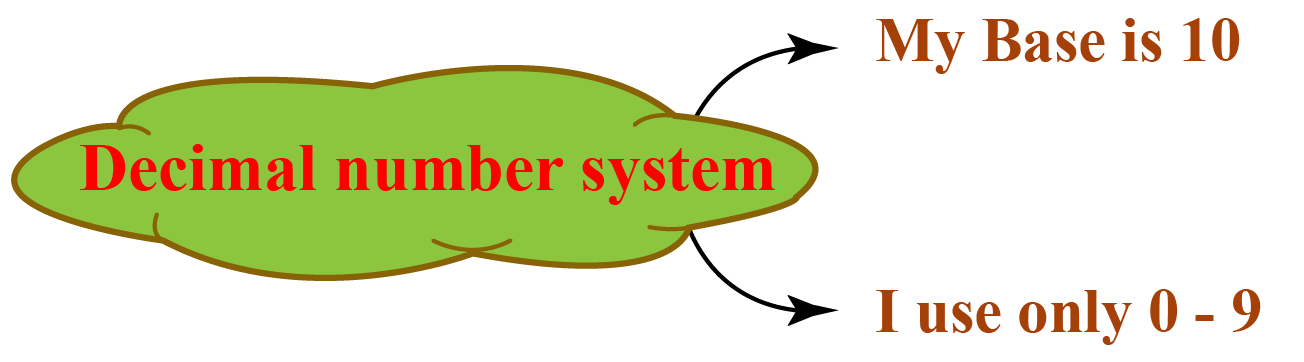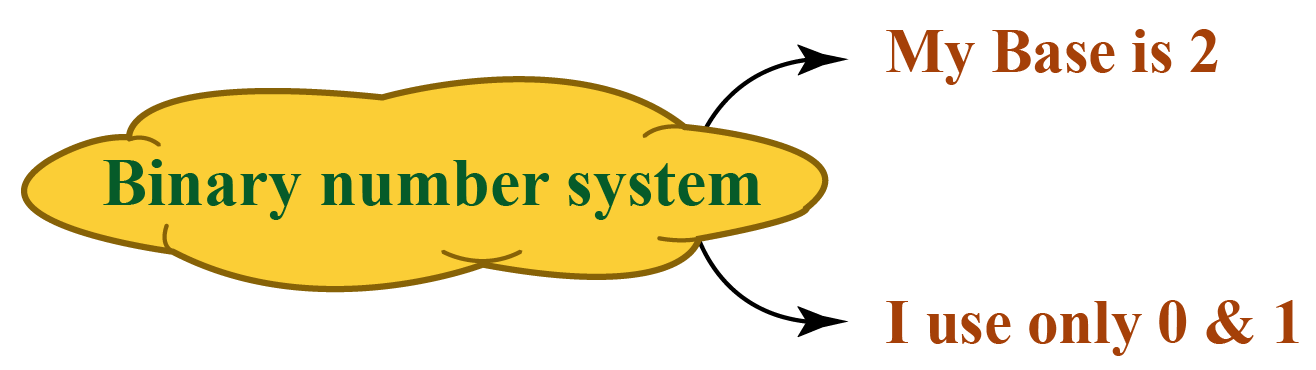$$64_{10} \text{ in binary is } 1000000_{2}$$

## How to Convert 64 From Decimal to Binary?

Let us look at the steps showing the conversion of 64 from decimal to binary.

Step 1:- Identify the base of the required number. In this case, the base of $$64$$ is $$10$$, i.e., $$64_{10}$$

Step 2:- Divide the given number 64 by the base (2) and note down the quotient and the remainder in the “quotient-remainder” form.

Repeat this process (dividing the quotient again by the base) until we get the quotient to be less than 2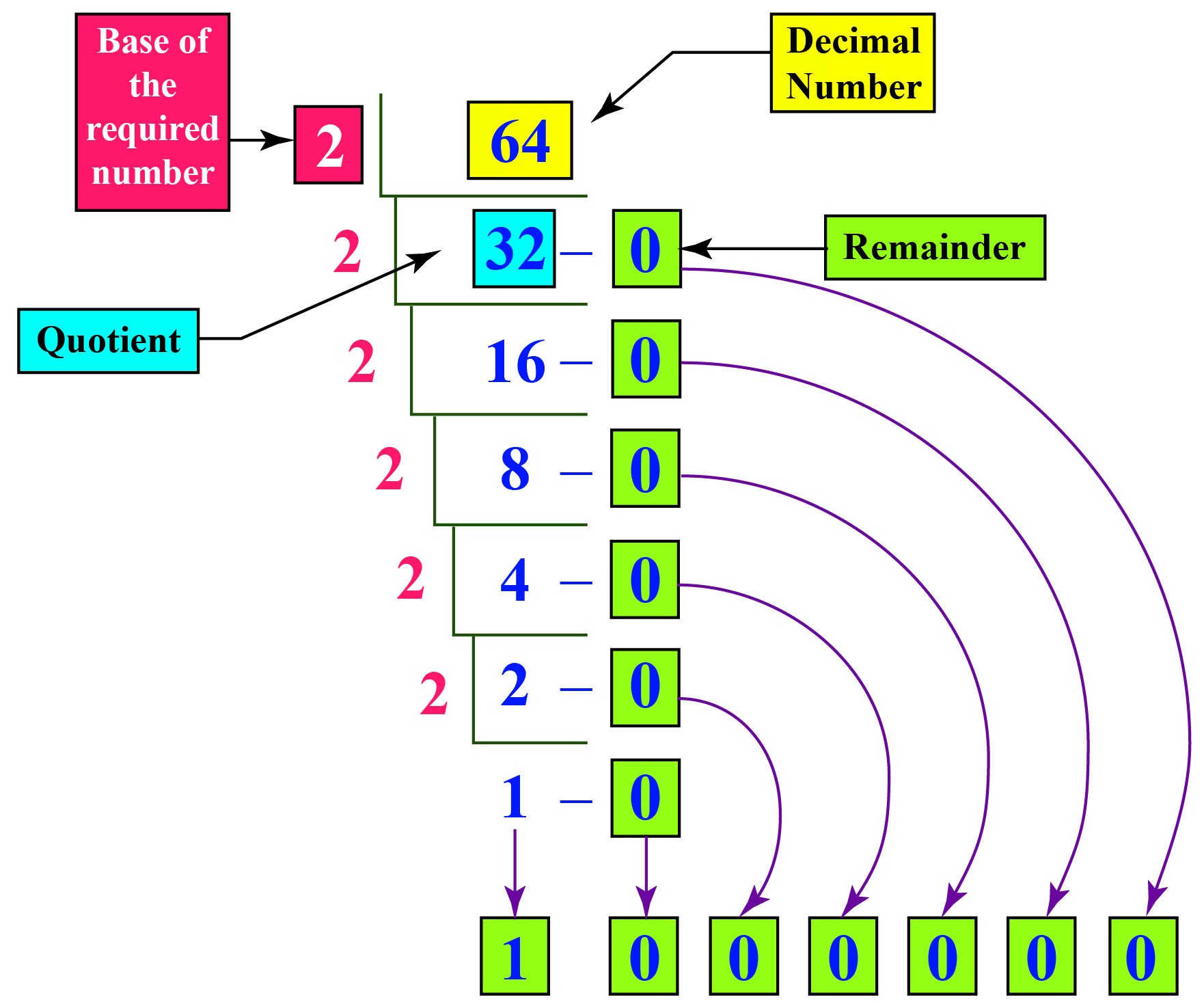Therefore  $$64_10 = 1000000_2$$

Convert 64 from Binary to Decimal

Converting $$1000000_2$$ to decimal

Step 1: Identify the base of the given number.

Here, the base of $$1000000_2$$ is 2

Step 2: Multiply each digit of the given number, starting from the rightmost digit, with the exponents of the base.

The exponents should start with $$0$$ and increase by $$1$$ every time we move from right to left.

Since the base here is $$2$$, we multiply the digits of the given number by $$2^0, 2^1, 2^2,...$$ from right to left.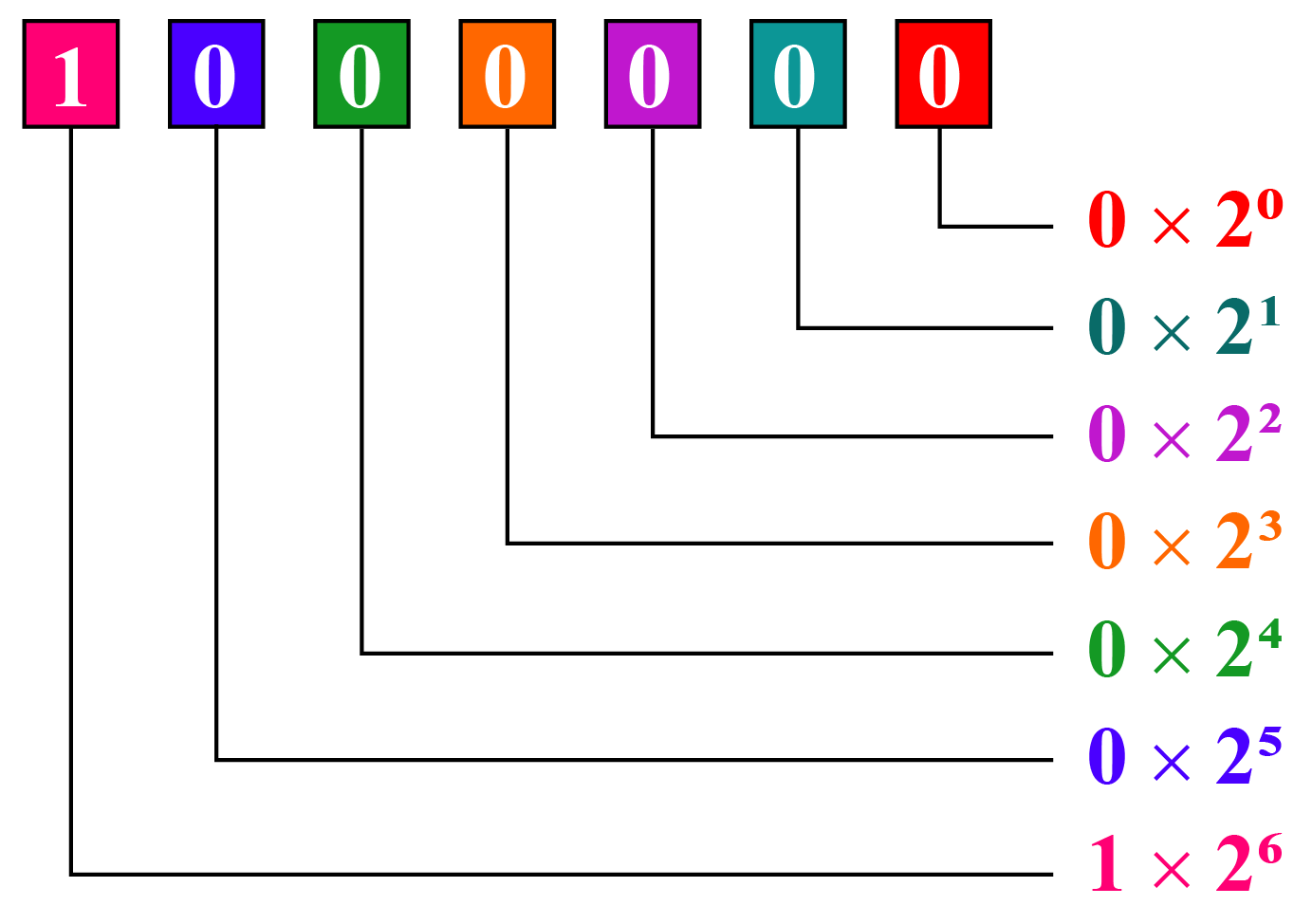Step 3: We just simplify each of the above products and add them.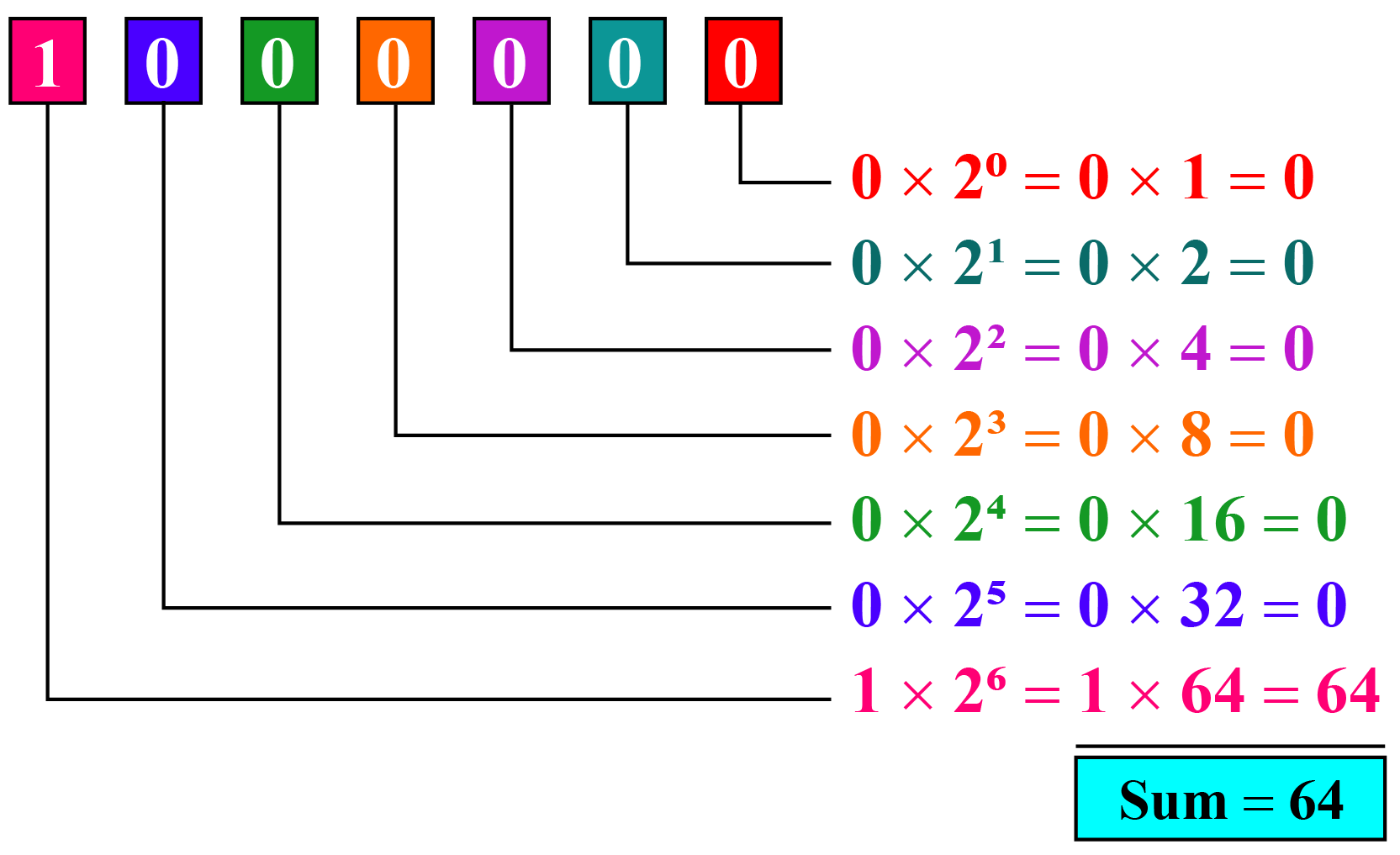Here, the sum is the equivalent number in the decimal number system of the given number.

 $$\therefore 1000000_2 = 64_{10}$$

More Important Topics
Numbers
Algebra
Geometry
Measurement
Money
Data
Trigonometry
Calculus